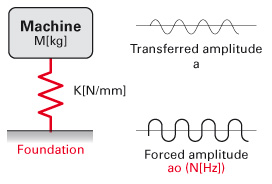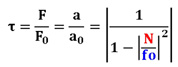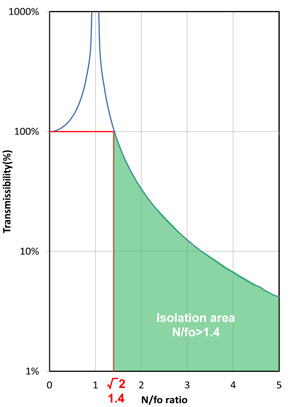1. Home
2. Products
3. Vibration Isolation Rubber
4. Introduction and theory
5. Design theory of VIR
1. Text Size
2. Small
3. Medium
4. Large

# Design theory of Vibration Isolation Rubber

When we study vibration isolation design, we approach it from two directions.

1. Active application: Reducing vibration from the machine to the ground
2. Passive application: Reducing vibration from the ground to the machineActive Application
(example: Engine mounting system)Passive Application
(example: Cabin mounting system)

When part of a machine is supported by vibration isolation rubber we define the machine's excitation force as "f0" and the excitation amplitude of the ground as "a0". The transmitted force to the ground is defined as "F" and the transmitted amplitude to the machine as "a". We express this phenomenon by following an "F1" formula called "vibration transmissibility".

## Vibration transmissibilityF1：Vibration Transmissibility Formula
N: Interference frequency (Hz)
fo: Natural frequency (Hz)

Figure.1 is a “vibration transmissibility curve". Vibration transmissibility is determined by the ratio between excitation frequency (=N Hz) and natural frequency (=fo Hz). If this value (N/fo ratio) becomes 1, vibration will be resonated and amplified to an unlimited degree. In order to create an isolation effect, we carefully select the spring stiffness of the vibration isolation rubber and tune the N/fo ratio above 1.4.Figure 1. Magnification factor

## Natural frequency

Natural frequency (=fo) can be calculated by the following “F2” formula.F2：Natural Frequency Formula
Fo: Natural frequency (Hz)
K: Dynamic spring stiffness (N/mm)
M: Weight of supported object (Kg)

It also can be expressed as shown in the graph (Figure.2).

If we know the static support weight and targeted natural frequency, we can project the required spring stiffness of a vibration isolation rubber mount using the graph below. (Generally speaking, the dynamic spring stiffness of a vibration isolation rubber mount is 1.2 to 1.4 times static spring stiffness.)Figure 2. Natural freqency (fo) chart

Example: Using the above graph, if the supporting weight per mount is 100kg and targeted natural frequency (fo) is 10Hz, the required dynamic spring stiffness of the vibration isolation rubber mount becomes 400N/mm. The Dynamic Spring Stiffness of rubber is typically 1.2 to 1.4 higher than static spring stiffness,. So in this case, the required static spring stiffness range is approximately 285 to 330N/mm.